﻿ 光纤光栅应变传感器测量应变误差分析 Error Analysis of Measured Strain of Fiber Bragg Grating Strain Sensor

Instrumentation and Equipments
Vol. 07  No. 01 ( 2019 ), Article ID: 29358 , 8 pages
10.12677/IaE.2019.71009

Error Analysis of Measured Strain of Fiber Bragg Grating Strain Sensor

Dianliang Sun1, Rujun Wu2, Xiaofeng Zhang2, Tian Chen2, Xuefen Ma2

1ShanDong Post and Telecom Engineering CO. LTD., Ji’nan Shandong

2School of Mechanical Engineering, Shanghai Dianji University, Shanghai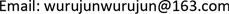Received: Feb. 25th, 2019; accepted: Mar. 13th, 2019; published: Mar. 21st, 2019ABSTRACT

The strain measured by FBG (Fiber Bragg Grating) sensors is not equal to the true strain. To improve the measurement accuracy, the relationship between the measured strain and the real strain needs to be studied. Based on the theoretical analysis, the strain measurement error caused by the lateral effect is obtained. For the complex plane strain problem, the calculation formula of the measured strain was revised, and the effects of the installation angle, installation deviation angle on the measurement accuracy were analyzed. The analysis shows that the relative error of strain measurement is approximately sinusoidal to the installation angle. The relative error of strain measurement gradually decreases with the increase of the principal strain ratio. This theory has a high accuracy, and provides theoretical guidance for the application of fiber gratings.

Keywords:Lateral Effect, Strain, Installation Deviation, Measurement Accuracy

1山东省邮电工程有限公司，山东 济南

2上海电机学院，机械学院，上海1. 引言

2. 考虑横向效应的应变测量

2.1. 光纤光栅的横向效应

$K{\epsilon }_{x}=\Delta \lambda /\lambda$ (1)

$H={K}_{B}/{K}_{L}$ (2)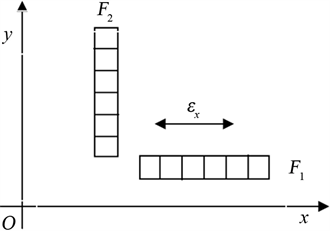Figure 1. Measurement of transverse coefficients

2.2. 平面应变场的应变测量分析

$\left\{\begin{array}{l}{\epsilon }_{1}={l}_{1}^{2}{\epsilon }_{x}+{m}_{1}^{2}{\epsilon }_{y}+{l}_{1}{m}_{1}{\gamma }_{xy}\\ {\epsilon }_{2}={l}_{2}^{2}{\epsilon }_{x}+{m}_{2}^{2}{\epsilon }_{y}+{l}_{2}{m}_{2}{\gamma }_{xy}\\ {\epsilon }_{3}={l}_{3}^{2}{\epsilon }_{x}+{m}_{3}^{2}{\epsilon }_{y}+{l}_{3}{m}_{3}{\gamma }_{xy}\end{array}$ (3)

$\left\{\begin{array}{l}{\epsilon }_{x}={\epsilon }_{1}\\ {\epsilon }_{y}={\epsilon }_{2}\\ {\gamma }_{xy}=2{\epsilon }_{3}-{\epsilon }_{1}-{\epsilon }_{2}\end{array}$ (4)

$\Delta \lambda =\lambda {K}_{L}\left(1+\frac{H{\epsilon }_{B}}{{\epsilon }_{L}}\right)\cdot {\epsilon }_{L}=\lambda {K}_{L}\left(1+\alpha H\right)\cdot {\epsilon }_{L}$ (5)

$\Delta \lambda =\lambda {K}_{L}\left(1-H\mu \right)\cdot {\epsilon }_{L}$ (6)

$\Delta \lambda =\lambda {K}_{L}\left(1+\alpha H\right)\cdot {\epsilon }_{L}=\lambda {K}_{L}\cdot {\epsilon }_{m}$ (7)

${\epsilon }_{m}={\epsilon }_{L}\left(1+\alpha H\right)$ (8)

$e=\frac{{\epsilon }_{m}-{\epsilon }_{L}}{{\epsilon }_{m}}=\frac{\alpha H}{1+\alpha H}$ (9)

$\left\{\begin{array}{l}\Delta {\lambda }_{1}=\lambda {K}_{L}\left(1+\frac{{\epsilon }_{2}}{{\epsilon }_{1}}H\right)\cdot {\epsilon }_{1}\\ \Delta {\lambda }_{2}=\lambda {K}_{L}\left(1+\frac{{\epsilon }_{1}}{{\epsilon }_{2}}H\right)\cdot {\epsilon }_{2}\\ \Delta {\lambda }_{3}=\lambda {K}_{L}\left(1+\frac{{\epsilon }_{4}}{{\epsilon }_{3}}H\right)\cdot {\epsilon }_{3}\end{array}$ (10)

$\left\{\begin{array}{l}{\epsilon }_{x}={\epsilon }_{1}=\frac{\Delta {\lambda }_{1}-\Delta {\lambda }_{2}H}{\lambda {K}_{L}\left(1-{H}^{2}\right)}\\ {\epsilon }_{y}={\epsilon }_{2}=\frac{\Delta {\lambda }_{2}-\Delta {\lambda }_{1}H}{\lambda {K}_{L}\left(1-{H}^{2}\right)}\\ {\gamma }_{xy}=\frac{2\Delta {\lambda }_{3}-\Delta {\lambda }_{1}-\Delta {\lambda }_{2}}{\lambda {K}_{L}\left(1-H\right)}\end{array}s$ (11)

3. 安装偏差对测量应变的影响

${\epsilon }_{\phi }=\frac{{\epsilon }_{1}+{\epsilon }_{2}}{2}+\frac{{\epsilon }_{1}-{\epsilon }_{2}}{2}\mathrm{cos}2\phi$ (12)

${\epsilon }_{测}=\frac{{\epsilon }_{1}+{\epsilon }_{2}}{2}+\frac{{\epsilon }_{1}-{\epsilon }_{2}}{2}\mathrm{cos}2\left(\phi +\Delta \phi \right)$ (13)

$\Delta {\epsilon }_{\phi }=\left({\epsilon }_{1}-{\epsilon }_{2}\right)\mathrm{sin}\left(2\phi +\Delta \phi \right)\mathrm{sin}\Delta \phi$ (14)

$e=\frac{\Delta {\epsilon }_{\phi }}{{\epsilon }_{\phi }}=\frac{2\left({\epsilon }_{1}-{\epsilon }_{2}\right)\mathrm{sin}\left(2\phi +\Delta \phi \right)\mathrm{sin}\Delta \phi }{2{\epsilon }_{1}+{\epsilon }_{2}-{\epsilon }_{2}\mathrm{cos}2\phi }$ (15)

$e=\frac{2\left(1-\alpha \right)\mathrm{sin}\left(2\phi +\Delta \phi \right)\mathrm{sin}\Delta \phi }{2+\alpha -\alpha \mathrm{cos}2\phi }$ (16)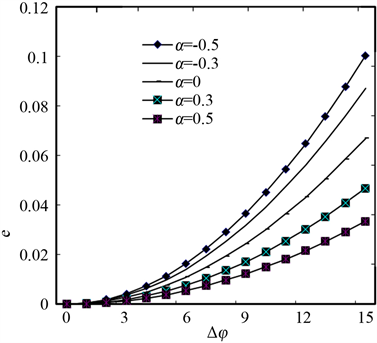Figure 2. The relationship between installation angle deviation and relative error of measured strain with $\phi ={0}^{\circ }$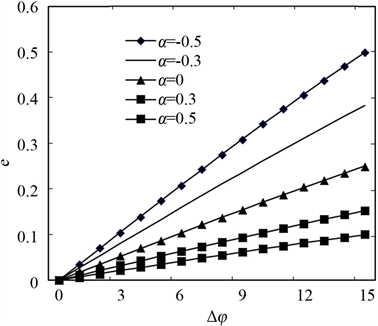Figure 3. The relationship between installation angle deviation and relative error of measured strain with $\phi ={45}^{\circ }$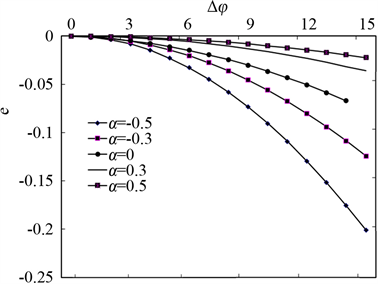Figure 4. The relationship between installation angle deviation and relative error of measured strain with $\phi ={90}^{\circ }$

$\begin{array}{c}e=\frac{\Delta {\epsilon }_{\phi }}{{\epsilon }_{\phi }}=\frac{2\left({\epsilon }_{1}-{\epsilon }_{2}\right)\mathrm{sin}\left(2\phi +\Delta \phi \right)\mathrm{sin}\Delta \phi }{2{\epsilon }_{1}+{\epsilon }_{2}-{\epsilon }_{2}\mathrm{cos}2\phi }\\ =\frac{2{\epsilon }_{1}\left(1+\mu \right)\mathrm{sin}\left(2\phi +\Delta \phi \right)\mathrm{sin}\Delta \phi }{2{\epsilon }_{1}-\mu {\epsilon }_{1}+\mu {\epsilon }_{1}\mathrm{cos}2\phi }\\ =\frac{2\left(1+\mu \right)\mathrm{sin}\left(2\phi +\Delta \phi \right)\mathrm{sin}\Delta \phi }{2-\mu +\mu \mathrm{cos}2\phi }\end{array}$

$\mu =0.3$，安装角度 $\phi$ 依次为0˚、30˚、45˚和90˚时，不同安装角度下的测量应变相对误差分布如图5所示，从图5中得出，测量应变相对误差随安装角度偏差角度的增大而变大，但是，在相同安装偏差角度下，安装角度在 $\phi ={45}^{\circ }$ 时的相对误差较大，在 $\phi ={0}^{\circ },{90}^{\circ }$ 时，相对误差比较小。以相对误差5%为基准，则安装偏差角度 $\phi$ 在0˚、30˚、45˚和90˚下的最大允许安装偏差角度依次为：3.5˚、3.9˚、2.3˚、1.9˚和3˚。

$\mu =0.3$，安装角度偏差 $\Delta \phi$ 依次为1˚、3˚、5˚和7˚时，相对误差随安装角度的变化如图6所示。从图6可以得出：相对误差随着安装角度成类似于正弦函数变化，在安装角为45˚附近最大，在0˚、90˚附近最小。所以在实际应变测量时，应该尽量是安装角度为0˚或90˚附近。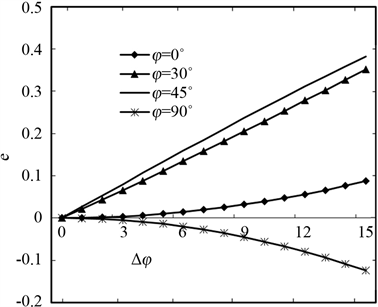Figure 5. Effect of installation angle on relative error of measured strain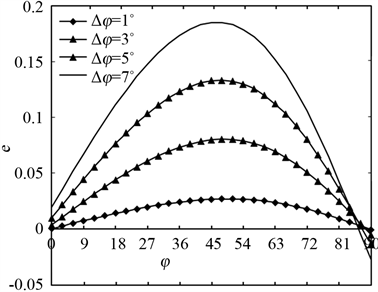Figure 6. Effect of installation angle deviation on relative error of measured strain

4. 结论

1) 详细推导了因横向效应而引起的应变测量误差，并进一步建立了考虑横向效应在内的复杂平面应变问题的应变测量修正公式。

2) 分析了由光纤光栅安装角度误差而产生测量误差的机理，并建立了角度误差与测量误差之间的关系表达式。

3) 在相同安装偏差角度下，应变相对误差与安装角度成近似正弦变化；安装偏差角度越大则相对误差越大；正应变比 $\alpha$ 越大，则测量误差越小。

Error Analysis of Measured Strain of Fiber Bragg Grating Strain Sensor[J]. 仪器与设备, 2019, 07(01): 58-65. https://doi.org/10.12677/IaE.2019.71009

1. 1. 长自佳. 光纤光栅理论基础与传感技术[J]. 北京: 科学技术出版社, 2009.

2. 2. 周雪芳. 光纤布拉格光栅振动传感技术研究[D]: [硕士学位论文]. 武汉: 武汉理工大学, 2003.

3. 3. 徐国权, 熊代余. 光纤光栅传感器技术在工程中的应用[J]. 中国光学, 2013, 6(3): 306-317.

4. 4. Zhou, J., Zhou, Z. and Zhang, D. (2010) Study on Strain Transfer Characteristics of Fiber Bragg Grating Sensors. Material Systems and Structures, 21, 1117-1122.

5. 5. 朱四荣, 等. 光纤布拉格光栅的横向效应研究[M]. 武汉: 武汉理工大学, 2005.

6. 6. 刘春桐, 陈平, 李洪才, 等. 光纤光栅横向效应在平面应变测量中的应用[J]. 光学与光电技术, 2008, 6(6): 29-32.

7. 7. 苏晨辉, 张雷, 隋青美, 等. 表面粘贴式光纤光栅传感器的应变传递机理分析与实验研究[J]. 传感技术学报, 2018, 31(4): 513-517.

8. 8. 张俊康, 孙广开, 李红, 等. 变形机翼薄膜蒙皮形状监测光纤传感方法研究[J]. 仪器仪表学报, 2018, 39(2): 66-72.

9. 9. 梅钰洁, 余有龙, 耿淑伟. 基于光纤光栅的螺纹管压弯扭测量技术[J]. 光子学报, 2017, 46(9): 167-172.

10. 10. 孙媛凯, 谢涛, 赵振刚, 等. 光纤Bragg光栅倾角传感器的标定与不确定度分析[J]. 光学技术, 2017, 43(3): 252-255.

11. 11. 徐芝纶. 弹性力学[M]. 北京: 高等教育出版社, 2006.

12. 12. 王守新. 材料力学[M]. 大连: 大连理工大学出版社, 2006.